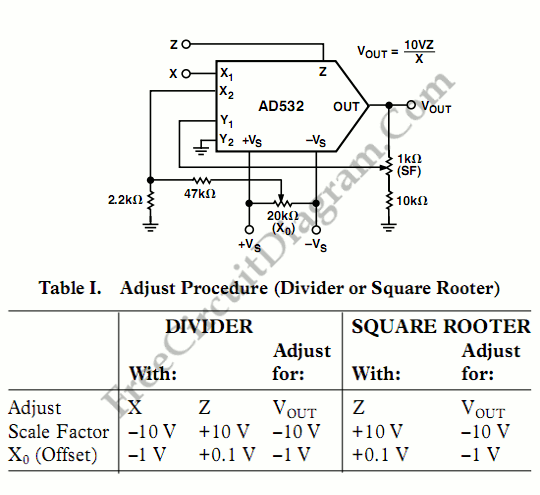# Single Chip Divider CircuitWe can build a divider circuit using only a single chip component, a special purpose integrated circuit AD532. We can configure the AD532 as a two quadrant divider by connecting the multiplier cell in the feedback loop of the op amp and using the Z terminal as a signal input, as shown in the following figure. For your note, the output error is given approximately by 10 Vεm/ (X1-X2), where εm is the total error specification for the multiply mode and bandwidth by fm x (X1-X2)/10 V, where fm is the bandwidth of the multiplier. The X input is restricted to negative values to avoid positive feedback. Thus, connect the input to X and the offset null to X2 for single ended negative inputs. For single ended positive inputs (0V to +10V), connect the input to X2 and the offset null to X1. Gain (S.F) and offset (X0) adjustment are recommended as shown and explained in the following table for optimum performance. The useful range in denominator input is approximately 500 mV ≤ (X1-X2) ≤ 10 V for practical reason. If used, the voltage offset adjust (Vos) is trimmed with Z at zero and (X1-X2) at full scale.